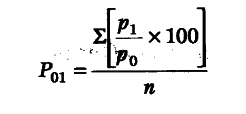# Discuss simple average of price relative method

Simple Average of Price Relative Method According to this method, first of all price relative are obtained for various items and then take simple average of all the price relative.

Price Relative = Current Year Price / Base Year Price X 100

We can find out price index number of the current year by using the following formulaHere, p_{ 1 } / p_{ 0 } X 100 = Price relative, N = Number of goods,p_{ 1 } = Current year’s price, p_{ 0 } = Base year’s value.